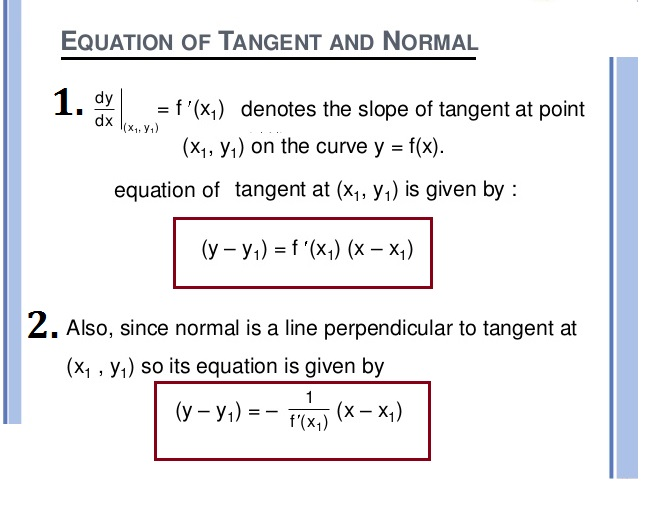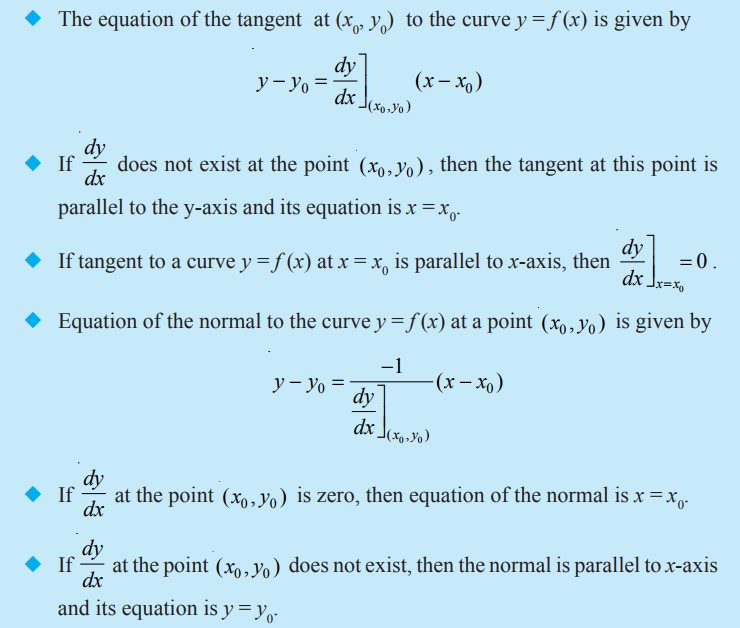Mathematics Tangents And Normals For CBSE-NCERT
Click for Only Video

### Topic covered

star Tangents And Normals

### Tangents and Normals\color{green} ✍️ The equation of a straight line passing through a given point (x_0, y_0) having finite slope m is given by

y – y_0 = m (x – x_0)

=> Note that the slope of the tangent to the curve y = f (x) at the point (x_0, y_0) is given by

color{red}{(dy)/(dx) ]_(x_0, y_0) = f' (x_0}. So

\color{green} ✍️ So The equation of the tangent at (x_0, y_0) to the curve y = f (x) is given by

color{green}{y – y_0 = f ′(x_0)(x – x_0)}

=>Also, since the normal is perpendicular to the tangent, the slope of the normal to the curve y = f (x) at (x_0, y_0) is

(-1)/(f'(x_0) ). if f' (x_0) ≠ 0 .

=> Therefore, the equation of Normal to the curve y = f (x) at (x_0, y_0)  is given by

color{green}{y - y_0 = (-1)/( f' (x_0 ) ) (x - x_0)}

i.e., color{green}{ (y- y_0) f' (x_0 ) + (x - x_0 ) = 0}

color{red}{"Key Concept :"} If a tangent line to the curve y = f (x) makes an angle θ with x-axis in the positive direction, then

color{blue}{(dy)/(dx) = "slope of the tangent" = tan θ}

### Particular cases

(i)\color{green} ✍️ If slope of the tangent line is zero, then tan θ = 0 and so θ = 0 which means the tangent line is parallel to the x-axis.

In this case, the equation of the tangent at the point (x_0, y_0) is given by color{green}{y = y_0}.

(ii) If θ -> pi/2, then tan θ→∞, which means the tangent line is perpendicular to the x-axis, i.e., parallel to the y-axis.

In this case, the equation of the tangent at (x_0, y_0) is given by color{green}{x = x_0}.

### Summery :Q 3115345269Find the slope of the tangent to the curve y = x^3 – x at x = 2.
Class 12 Chapter 6 Example 14Solution:

The slope of the tangent at x = 2 is given by

 (dy)/(dx) ]_(x=2) = 3x^2 -1 ]_(x=2) = 11 .
Q 3125445361Find the point at which the tangent to the curve y = sqrt (4x-3) -1 has its slope 2/3
Class 12 Chapter 6 Example 15Solution:

Slope of tangent to the given curve at (x, y) is

 (dy)/(dx) = 1/2 (4x-3)^(-1/2) 4 = 2/(sqrt (4x-3) )

The slope is given to be 2/3

So 2/(sqrt (4x-3) ) = 2/3

or 4x-3 = 9

or x= 3

Now y = sqrt (4x-3) -1 . So when x = 3, y = sqrt (4(3) -3) -1= 2 .

Therefore, the required point is (3, 2).
Q 3155445364Find the equation of all lines having slope 2 and being tangent to the curve

y + 2/(x-3) = 0
Class 12 Chapter 6 Example 16Solution:

Slope of the tangent to the given curve at any point (x,y) is given by

 (dy)/(dx) = 2/((x-3)^2)

But the slope is given to be 2. Therefore

2/((x-3)^2) = 2

or  (x-3)^2 = 1

or  x-3 = pm 1

or x= 2,4

Now x = 2 gives y = 2 and x = 4 gives y = – 2. Thus, there are two tangents to the
given curve with slope 2 and passing through the points (2, 2) and (4, – 2). The equation
of tangent through (2, 2) is given by
y – 2 = 2(x – 2)
or y – 2x + 2 = 0
and the equation of the tangent through (4, – 2) is given by
y – (– 2) = 2(x – 4)
or y – 2x + 10 = 0
Q 3155845764Find the equation of the tangent to the curve  y = (x-7)/( ( x-2)(x-3) ) at the point where it cuts the x-axis.
Class 12 Chapter 6 Example 18Solution:

Note that on x-axis, y = 0. So the equation of the curve, when y = 0, gives
x = 7. Thus, the curve cuts the x-axis at (7, 0). Now differentiating the equation of the
curve with respect to x, we obtain

 (dy)/(dx) = (1-y (2x-5) )/( (x-2)(x-3) )  (Why?)

or  (dy)/(dx)]_(7,0) = (1-0)/((5)(4)) = 1/20

Therefore, the slope of the tangent at (7, 0) is 1/20 . Hence, the equation of the
tangent at (7, 0) is

 y- 0 = 1/20 (x-7) or 20y − x + 7 = 0
Q 3125845761Find points on the curve  x^2/4 + y^2/25 =1 at which the tangents are (i) parallel to x-axis (ii) parallel to y-axis.
Class 12 Chapter 6 Example 17Solution:

Differentiating x^2/4 + y^2/25 =1 with respect to x, we get

x/2 + (2y)/(25) (dy)/(dx) = 0

or  (dy)/(dx) = (-25)/4 x/y

(i) Now, the tangent is parallel to the x-axis if the slope of the tangent is zero which

gives  (-25)/4 x/y = 0 . This is possible if x = 0. Then x^2/4 + y^2/25 =1 for x = 0 gives

y^2 =25 i.e., y = pm 5

Thus, the points at which the tangents are parallel to the x-axis are (0, 5) and
(0, – 5).

(ii) The tangent line is parallel to y-axis if the slope of the normal is 0 which gives

 (4y)/(25 x) = 0 , i.e. y =0 Therefore , x^2/4 + y^2/25 = 1 for y = 0 gives x = ± 2. Hence, the

points at which the tangents are parallel to the y-axis are (2, 0) and (–2, 0).
Q 3175845766Find the equations of the tangent and normal to the curve x^2/3 + y^2/3 = 2 at (1,1)
Class 12 Chapter 6 Example 19Solution:

Differentiating x^(2/3) + y^(2/3) = 2 with respect to x, we get

2/3 x^(-1/3) + 2/3 y^(-1/3) (dy)/(dx) = 0

or  (dy)/(dx) = - (y/x)^1/3

Therefore, the slope of the tangent at (1, 1) is  (dy)/(dx)]_(1,1) = -1

So the equation of the tangent at (1, 1) is

y – 1 = – 1 (x – 1) or y + x – 2 = 0

Also, the slope of the normal at (1, 1) is given by

(-1)/( text (slope of the tangent at (1,1)) ) = 1

Therefore, the equation of the normal at (1, 1) is

y – 1 = 1 (x – 1) or y – x = 0
Q 3105845768Find the equation of tangent to the curve given by

x = a sin^3 t , y = b cos^3 t ... (1)

at a point where  t = pi/2 .
Class 12 Chapter 6 Example 20Solution:

Differentiating (1) with respect to t, we get

(dx)/(dt) = 3 a sin^2 t cos t  and  (dy)/(dt) = -3 b cos^2 t sin t

or  (dy)/(dx) = ( (dy)/(dt) )/( (dx)/(dt) ) = (-3 b cos^2 t sin t)/(3 a sin^2 t cos t ) = (-b)/a (cos t )/( sin t )

Therefore, slope of the tangent at  t = pi/2 i

(dy)/(dx)]_(t = pi/2) = (-b cos pi/2 )/( a sin pi/2) =0

Also, when  t = pi/2 , x = a and y = 0 . Hence, the equation of tangent to the given

curve at t = pi/2 , i.e. at  (a, 0) is

y – 0 = 0 (x – a), i.e., y = 0.